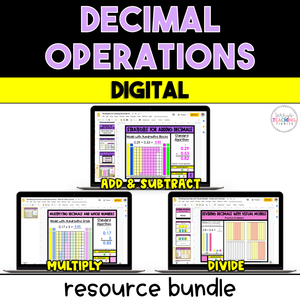# Decimal Operations Resource Bundle - Digital

• \$24.00
Unit price per
• Save \$4.50
Shipping calculated at checkout.

Use visual models along with the standard algorithm and area models to quickly and effectively teach your students how to add, subtract, multiply, and divide decimals with this comprehensive digital resource pack.

With 11 mini-lessons, (2 adding and subtracting decimals, 3 multiplying decimals, and 6 dividing decimals - 5 using the standard algorithm and one with visual models) you will have plenty of resources to teach your student how to solve problems using decimal operations.

Using visual models, students will have a more hands-on approach to “see” what it looks like to add, subtract, and multiply decimals as well as divide decimals using tenths and hundredths.  These models will help reinforce the use and understanding of the standard algorithm.  It’s great for kinesthetic learners as well as students that may struggle with memorizing algorithms.

This resource is complete, comprehensive, and easy to follow with a clear format.  It is low-prep, easy to assign to a sub, and contains engaging digital activities for use with Google Classroom.  If your students are struggling conceptually, this resource is for you!

Supports Common Core Standards (CCSS):  5.NBT.7

This resource is also included in a 5th Grade Math Year-Long Curriculum Bundle.

How Can You Use This?

Introduce each skill with the detailed set of notes and sample problems in Google Slides.  I suggest doing this in a whole group setting and pulling the slides up on a Smartboard if you’re in person or share the screen online if you’re teaching virtually.

Gradually release students to practice independently using the practice slides.

When all skills have been taught, provide additional practice and review using the set of digital task cards, then assess with the final quizzes when you feel students are ready.

WHAT OTHER TEACHERS ARE SAYING…

⭐️⭐️⭐️⭐️⭐️  This helped me differentiate my groups and worked well in breakout rooms during distance learning.  - Beverly M.

⭐️⭐️⭐️⭐️⭐️   I love all of the practice that is included in these. Thanks so much!  - Melissa B.

⭐️⭐️⭐️⭐️⭐️   This has been a great resource to use virtually.  - Julie D.

## What You Get

Mini-Lessons Included:

• Subtracting Decimals
• Multiplying Decimals by Whole Numbers
• Multiplying Decimals by Decimals
• Multiplying Decimals Using Area Models
• Dividing Decimals by Whole Numbers
• Dividing Whole Numbers by Decimals
• Dividing Decimals by Decimals (with the same # of decimal places in the dividend and divisor)
• Dividing Decimals by Decimals (with a different # of decimal places in the dividend and divisor)
• Dividing Decimals by Decimals in which you have to annex a zero in the dividend
• Dividing Decimals by Decimals using a visual model

What You’ll Get in EACH of the mini-lessons: